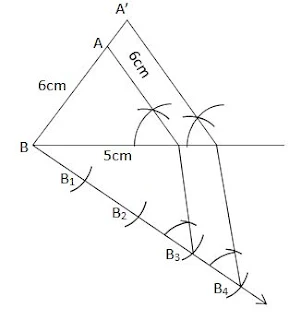## Revision Notes for Ch 11 Construction Class 10th Mathematics

Division of a line segment in a given ratio

Q. Draw Line segment PQ=9cm and divide it in the ratio 2:5. Justify your construction.

Steps of construction:

(i) Draw Line Segment PQ=9cm

(ii) Draw a Ray PX, making an acute angle with PQ.

(iii) Mark 7 points A1 , A2 , A3 …A7 along PX such that PA1 = A3A2 = A2A3 = A3A4 = A4A5 = A5A6 = A6A7

(iv) Join QA7

(v) Through the point A2 , draw a line parallel to A7Q by making an angle equal to ∠PA7Q at A2, intersecting PQ at point R. PR:RQ = 2:5

Justification:

We have A2R || A7QConstruction of a triangle similar to a given triangle as per the given scale factor when Scale Factor is less than 1

Q. Draw a ΔABC with sides BC = 8 cm, AC = 7 cm, and ÐB = 70°. Then, construct a similar triangle whose sides are (3/5)th of the corresponding sides of the ΔABC.

Steps of construction:
(i) Draw BC = 8 cm

(ii) At B, draw ∠XBC = 70°

(iii) With C as centre and radius 7 cm, draw an arc intersecting BX at A.

(iv) Join AB, and DABC is thus obtained.

(v) Draw a ray , making an acute angle with BC.

(vi) Mark 5 points, B1, B2, B3, B4, B5, along BY such that

(vii) BB1 = B1B2 = B2B3 = B3B4 = B4B5

(viii) Join CB5

(ix) Through the point B3, draw a line parallel to B5C by making an angle equal to ∠BB5C, intersecting BC at C´.

(x) Through the point C´, draw a line parallel to AC, intersecting BA at A´. Thus, ΔA´BC´ is Required Triangle.JustificationUsing BPT

Construction of a triangle similar to a given triangle as per the given scale factor when Scale Factor is more than 1
Q. Construct an isosceles triangle with base 5 cm and equal sides of 6 cm. Then, construct another triangle whose sides are of the corresponding sides of the (4/3)th of first triangle .

Steps of construction:
(i) Draw BC = 5 cm

(ii) With B and C as the centre and radius 6 cm, draw arcs on the same side of BC, intersecting at A.

(iii) Join AB and AC to get the required ΔABC.

(iv) Draw a ray , making an acute angle with BC on the side opposite to the vertex A.

(v) Mark 4 points B1, B2, B3, B4, along BX such that BB1 = B1B2 = B2B3 = B3B4

(vi) Join B3C. Draw a line through B4 parallel to B3C, making an angle equal to ∠BB3C intersecting the extended line segment BC at C´.

(vii) Through point C´, draw a line parallel to CA, intersecting extended BA at A´.

(viii) The resulting ΔA´BC´ is the required triangle.Construction of tangents to a circle
Q. Draw a circle of radius 3 cm. From a point 5 cm away from its centre, construct a pair